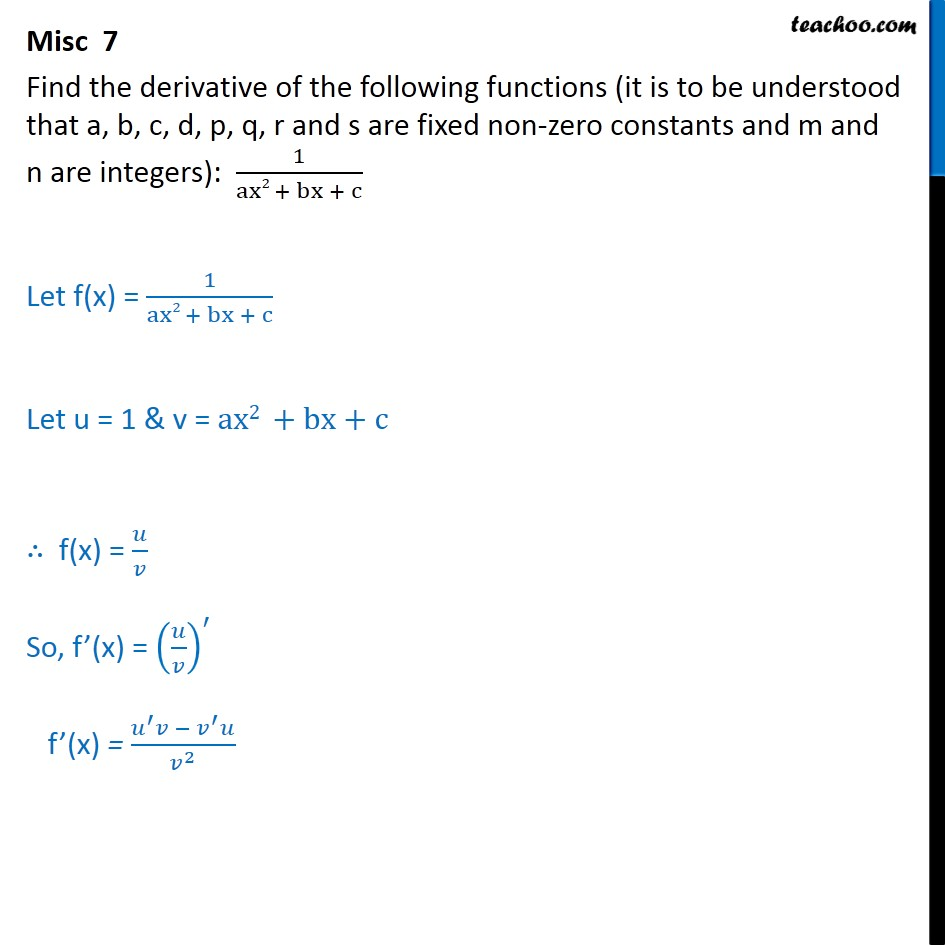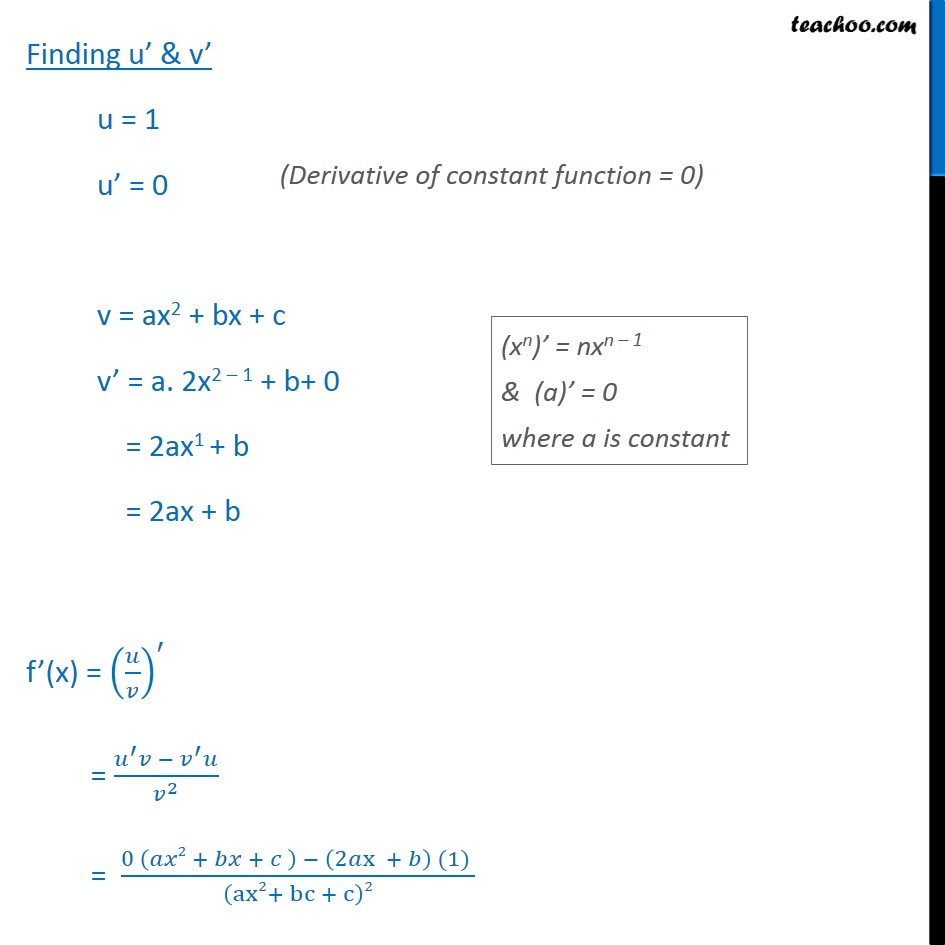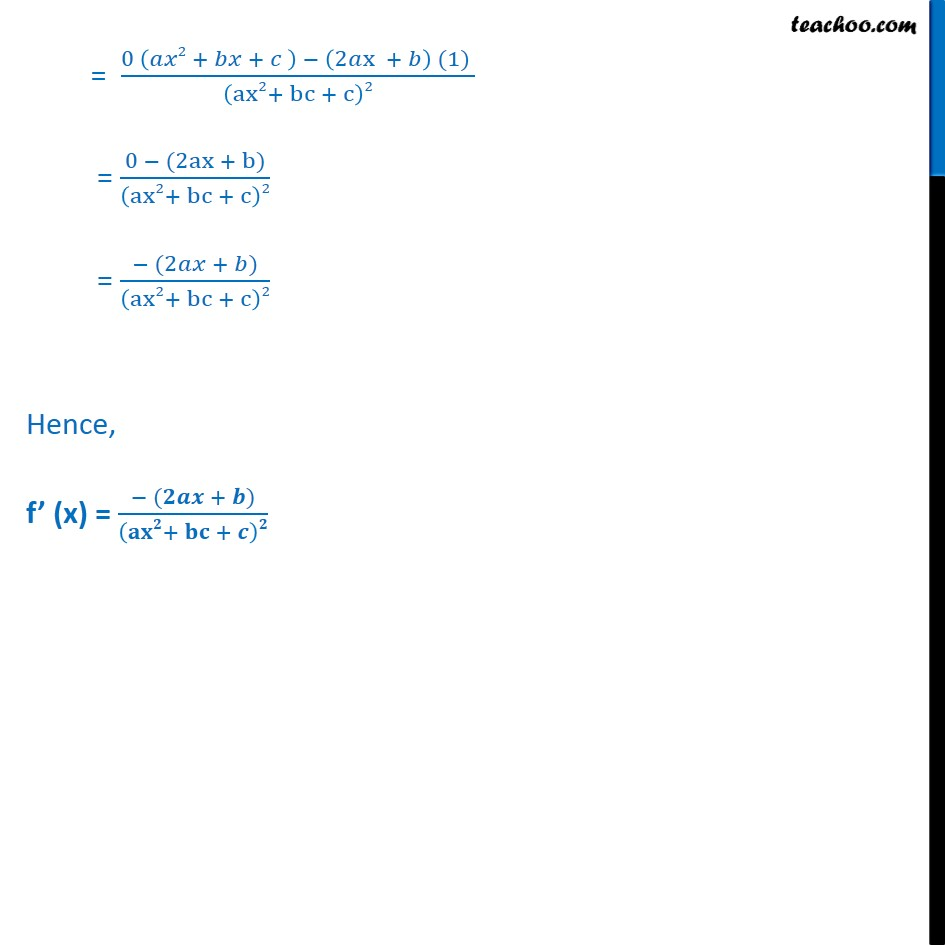Miscellaneous

Chapter 12 Class 11 Limits and Derivatives
Serial order wiseLearn in your speed, with individual attention - Teachoo Maths 1-on-1 Class

### Transcript

Misc 7 Find the derivative of the following functions (it is to be understood that a, b, c, d, p, q, r and s are fixed non-zero constants and m and n are integers): 1﷮ax2 + bx + c﷯ Let f(x) = 1﷮ax2 + bx + c﷯ Let u = 1 & v = ax2 +bx+c ∴ f(x) = 𝑢﷮𝑣﷯ So, f’(x) = 𝑢﷮𝑣﷯﷯﷮′﷯ f’(x) = 𝑢﷮′﷯𝑣 − 𝑣﷮′﷯𝑢﷮ 𝑣﷮2﷯﷯ Finding u’ & v’ u = 1 u’ = 0 v = ax2 + bx + c v’ = a. 2x2 – 1 + b+ 0 = 2ax1 + b = 2ax + b f’(x) = 𝑢﷮𝑣﷯﷯﷮′﷯ = 𝑢﷮′﷯𝑣 − 𝑣﷮′﷯𝑢﷮ 𝑣﷮2﷯﷯ = 0 𝑎𝑥2 + 𝑏𝑥 + 𝑐 ﷯ − 2𝑎x + 𝑏﷯ (1) ﷮ ax2+ bc + c﷯2﷯ = 0 𝑎𝑥2 + 𝑏𝑥 + 𝑐 ﷯ − 2𝑎x + 𝑏﷯ (1) ﷮ ax2+ bc + c﷯2﷯ = 0 − (2ax + b)﷮ ax2+ bc + c﷯2﷯ = − (2𝑎𝑥 + 𝑏)﷮ ax2+ bc + c﷯2﷯ Hence, f’ (x) = − (𝟐𝒂𝒙 + 𝒃)﷮ 𝐚𝐱𝟐+ 𝐛𝐜 + 𝒄﷯𝟐﷯